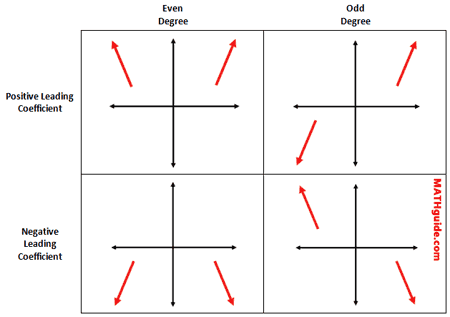# Unit 2!

` On this page we will discuss if a specific function is a polynomial or not, what makes it a polynomial, how to write the function in standard form, finding the degree, the type, the leading coefficient, and end behavior.   `

#### Step 1: Write the function

f(x) = 6x^3 -25x + 20; x = 5

#### Step 2: Determine if the funciton is a polynomial

` A function is a polynomial function if the degree of the exponents are positive whole numbers, if there is no square root, and if the function isnt being divided by x `

f(x) = 6x^3 -25x + 20; x = 5: this function in particular is a polynomial; given the previous rules

#### Step 3: Writing in standard form

To write a polynomial function in standard form, we must put our variables in order based on the degree

our function: f(x) = 6x^3 -25x + 20; x = 5, our function in standard form: f(x) = 6x^3 -25x^1 + 20x^0; x = 5

#### Step 4: Finding the Degree

The degree of our polynomial is determined by the biggest exponent in function

Degree of our function: 3, cubic

#### Step 5: Type of function

The type of function is determined by the degree of our function

Our degree: 3, so the type is cubic

```degree   name

1     linear (or monic)

3     cubic

4     quartic

5     quintic

6     hexic

7     septic

8     octic

9     nonic

10     decic  ```` given the information on this image, we know that the end behavior of our function is x - infinity, y + infinity, x + infinity, y + infinity. `